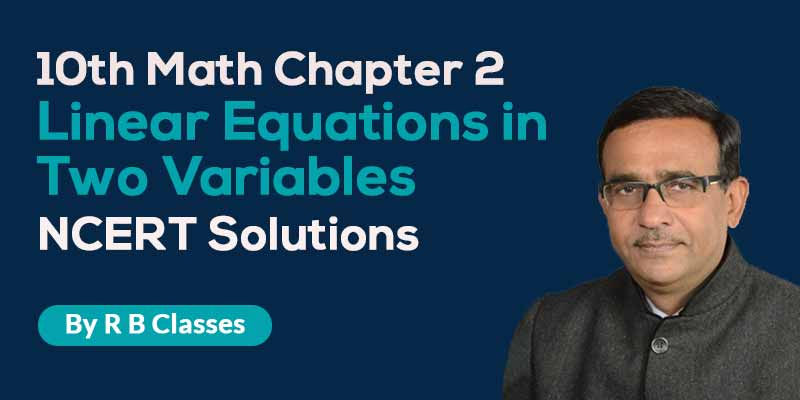## by R B Gautam

4.7 (3544 Users)
Class 10 , Maths
Board: CBSE & State Boards , Language: Hinglish

Mode- Online
Videos- 19
Validity- Till 31 Mar 2020
Language- Hinglish
No. of Views- Unlimited

## WHAT WILL I LEARN?

What will Student get?

• Class 10th Maths Linear Equations in Two Variables
• NCERT Exemplar Solution
• Important Questions.

## Curriculum

10th Maths Ch.3- Linear Equations in Two Variables

• 1. Q1 Solve the pair of linear equations by graphically
• 2. Q2 Distance, Speed & Time Word Problem
• 3. Q3 Find values of a and b if equations has infinitely many solution
• 4. Q4 Find the speed of the boat in still water and the speed of the stream
• 5. Q5 Class 10 maths linear equations
• 6. Q6 Linear equations in two variables word problems
• 7. Q7 Elimination method for solving system of equations
• 8. Pair of Linear Equations Important Questions Q1
• 9. Pair of Linear Equations(Substitution Method ) Important Questions NCERT Q2
• 10. Word problems on linear equations in two variables Important Question Q3
• 11. Linear Equations in two variables Important questions NCERT Q4
• 12. Solution of linear Equations by Cross Multiplication Method Q5
• 13. Linear Equations in two variable NCERT Q6
• 14. Find the value of a and b if Equations has infinite many solution Q7
• 15. Word Problems in Linear Equation IMP Question NCERT Q8
• 16. Linear Equations In Two Variables
• 17. Linear Equations In Two Variables ,for what value of k do the equations
• 18. Linear Equations In Two Variables, solve the system of equations graphically
• 19. Linear Equations In Two Variables , linear equations by the elimination method.

## About R B Gautam### R B Gautam

Mathematics | Class 9th to 12th | 25+ Years Experience | R B Gautam Sir is a well-known for his expertise in Math’s. He is serving the society by providing students with quality content to achieve 100 out of 100 in their math’s exams. R B Gautam Sir helps students by solving and explaining major problems faced by students in their exams.

## Student's Feedback## 499 299

Includes 18 % GST

Mode- Online

Videos- 19

Validity- Till 31 Mar 2020

Language- Hinglish

No. of Views- Unlimited